# INTRODUCTION

Kepler's laws are three laws of physics that describe the motion of planets in the solar system. Although the laws were formulated by Johannes Kepler exclusively for planets orbiting the Sun, the laws are more general and describe the motion of any object in the central gravitational field. An example is the motion of a satellite around the Earth or a comet around the Sun. Decades later, Isaac Newton showed that Kepler's laws were a consequence of his theory of gravity and mechanics. In this part, all three of Kepler's laws will be formulated and their meaning explained.

Before we state Kepler's laws, let's dive into history for a moment. The work of Danish astronomer Tycho Brahe (1546 1601), who performed very accurate astronomical measurements of planetary positions for his period, in the second half of the 16th century, played a key role in formulating the laws of planetary motion. Fate decided that in 1599 he moved to the court of Emperor Rudolf II. situated in Prague, where he met and worked briefly (until his death in 1601) with the German astronomer and mathematician Johannes Kepler. Sometimes we talk about a meeting of the greatest experimenter and theorist of the period. By analysing Brahe's data, Kepler discovered the laws of motion of planets, which in Kepler's honour are called Kepler's laws.

There are many formulations of Kepler's laws that differ in their generality. First, we will list the most common formulations of laws, which we will gradually refine.

It will take some math and physics, which are not taught in primary school, to understand some of the connections. In this text we will get a quick explanation of powers and roots, we will describe the ellipse and from physics we will look at Newton's law of universal gravitation and centrifugal force.

# Power and root

At the beginning we will define the power and we will continue with motivational examples, where we will get to know the rules that apply to working with powers. We will follow up by talking about the root and its relation to the power. We illustrate how to write numbers in a scientific format with the help of a power and why powers and roots are important in physics.

## Definition of power

In math class, we use multiplication to write the sum of several identical numbers, for example: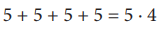The number "5" tells us what is repeated, and the number "4" how many times it does. Of course, one could argue that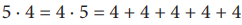is just another notation of the number 20. If we replace the addition by multiplication, it is a habit to write repeated multiplication with the same number in the form: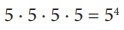The expression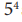is called the power (power expression), 5 is the base of the power and 4 is the exponent of the powers. The power tells us what multiplies and the exponent how many times it does.

We must realize that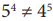, because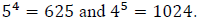More complex expressions can be abbreviated using power notation: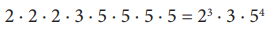Another example could be: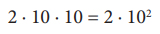this can also be written as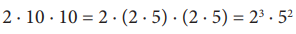It is worth mentioning, for example, that we would write the number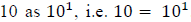. Furthermore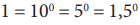and the like, which means that in the given expression, the number 10, resp. 5, resp. 1.5 occurs "zero times", i. e. it is not present at all.

## Considerations of selected properties of powers

We will try to illustrate the validity of these relations with examples: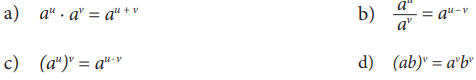where

filter_none